Courses

# Unit 1: Index Numbers (Part-2) CA Foundation Notes | EduRev

## Business Mathematics and Logical Reasoning & Statistics

Created by: Sushil Kumar

## CA Foundation : Unit 1: Index Numbers (Part-2) CA Foundation Notes | EduRev

The document Unit 1: Index Numbers (Part-2) CA Foundation Notes | EduRev is a part of the CA Foundation Course Business Mathematics and Logical Reasoning & Statistics.
All you need of CA Foundation at this link: CA Foundation

Summary

• An index number is a ratio or an average of ratios expressed as a percentage, Two or more time periods are involved, one of which is the base time period.
• Issues Involved in index numbers
(a ) Selection of Data
(b) Base period
(c) Selection of Weights:
(d) Use of Averages:
(e) Choice of Variables

Construction of Index Number
Price Index numbers
(a) Simple aggregative price index =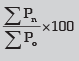(b) Laspeyres’ Index: In this Index base year quantities are used as weights:
Laspeyres Index =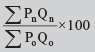(c) Paasche’s Index: In this Index current year quantities are used as weights:
Passche’s Index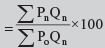(d) The Marshall-Edgeworth index uses this method by taking the average of the base year and the current year
Marshall-Edgeworth Index  =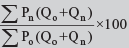• Fisher’s ideal Price Index: This index is the geometric mean of Laspeyres’ and Paasche’s.
Fisher’s Index =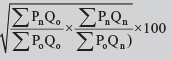• Weighted Average of Relative Method: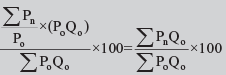• (h) Chain Index =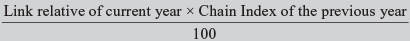Quantity Index Numbers:

• Simple aggregate of quantities: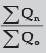• The simple average of quantity relatives: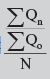• Weighted aggregate quantity indices:
(i) With base year weight :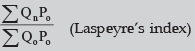(ii) With current year weight :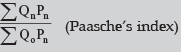(iii) Geometric mean of (i) and (ii) :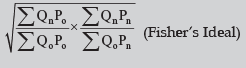• Base-year weighted average of quantity relatives. This has the formula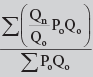• Value Indices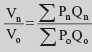• Deflated Value =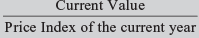or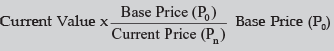• Shifted Price Index =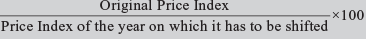(1) Unit test (2) Time reversal Test (3) Factor reversal test (4) Circular Test
Offer running on EduRev: Apply code STAYHOME200 to get INR 200 off on our premium plan EduRev Infinity!

69 docs|76 tests

,

,

,

,

,

,

,

,

,

,

,

,

,

,

,

,

,

,

,

,

,

;